#### 期刊菜单

Influence Law of Sun Wheel Bearing Support Stiffness on the Uniform Load Characteristics of Planetary Gear Transmission System
DOI: 10.12677/MET.2022.113023, PDF, HTML, XML, 下载: 49  浏览: 93  科研立项经费支持

Abstract: In this paper, the dynamics model of a single-row 2K-H planetary gear reducer is established, and its vibration differential equations are derived. Using the parameters of an experimental gearbox as simulation parameters, a simulation study was conducted on the influence law of support stiffness on the equal load characteristics of the planetary gear transmission system. It is found that the use of a smaller support stiffness of the sun wheel bearing can optimize the equal load characteristics of the internal meshing pair of the system, and a smaller support stiffness means that the center member has a certain floating effect, which can optimize the equal load characteristics.

1. 引言

1.1. 行星齿轮机构的力学模型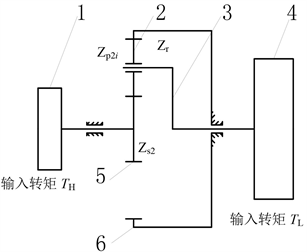Figure 1. Sketch of the transmission of planetary gear system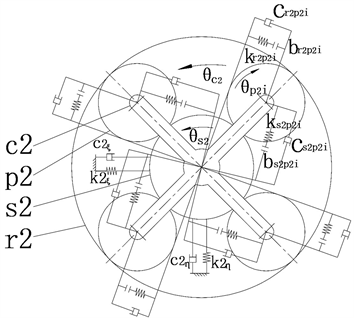Figure 2. Dynamics model of planetary gearing systemTable 1. Main parameters of the system

1.2. 试验用单排行星齿轮机构的运动微分方程Table 2. Main error parameters of the system

$\left\{\begin{array}{l}{e}_{s2pi}\left(t\right)={e}_{{a}_{s2pi}}\mathrm{sin}\left(\omega t+{\beta }_{s2i}\right)+{F}_{s2}\mathrm{sin}\left[{\omega }_{s2c}t+{\beta }_{s2}-\frac{2\pi \left(i-1\right)}{N}+\alpha \right]+{F}_{p2i}\mathrm{sin}\left({\omega }_{p2c}t+{\beta }_{p2i}+\alpha \right)\\ \text{\hspace{0.17em}}\text{\hspace{0.17em}}\text{\hspace{0.17em}}\text{\hspace{0.17em}}\text{\hspace{0.17em}}\text{\hspace{0.17em}}\text{\hspace{0.17em}}\text{\hspace{0.17em}}\text{\hspace{0.17em}}\text{\hspace{0.17em}}\text{\hspace{0.17em}}\text{\hspace{0.17em}}\text{\hspace{0.17em}}+{A}_{s2}\mathrm{sin}\left(-{\omega }_{c2}t+{\gamma }_{s2}+\alpha -\frac{2\pi \left(i-1\right)}{N}\right)+{A}_{p2i}\mathrm{sin}\left({\gamma }_{p2i}+\alpha \right)\\ {e}_{r2pi}\left(t\right)={e}_{{a}_{r2pi}}\mathrm{sin}\left(\omega t+{\beta }_{r2i}\right)+{F}_{p2i}\mathrm{sin}\left({\omega }_{p2c}t+{\beta }_{p2i}+\alpha \right)\\ \text{\hspace{0.17em}}\text{\hspace{0.17em}}\text{\hspace{0.17em}}\text{\hspace{0.17em}}\text{\hspace{0.17em}}\text{\hspace{0.17em}}\text{\hspace{0.17em}}\text{\hspace{0.17em}}\text{\hspace{0.17em}}\text{\hspace{0.17em}}\text{\hspace{0.17em}}\text{\hspace{0.17em}}\text{\hspace{0.17em}}-{F}_{r2}\mathrm{sin}\left[{\omega }_{r2c}t+{\beta }_{r2}-\frac{2\pi \left(i-1\right)}{N}+\alpha \right]+{A}_{p2i}\mathrm{sin}\left({\gamma }_{p2i}-\alpha \right)\end{array}$ (1)

${x}_{s2di}=-{\xi }_{s2}\mathrm{sin}\left({\phi }_{i}-\alpha \right)+{\eta }_{s2}\mathrm{cos}\left({\phi }_{i}-\alpha \right)$ (2)

${F}_{s2p2i}={k}_{s2p2i}f\left({x}_{s2p2i}+{x}_{s2di}-{e}_{s2p2i},{b}_{s2p2i}\right)$ (3)

${F}_{r2p2i}={k}_{r2p2i}f\left({x}_{r2p2i}-{e}_{r2p2i},{b}_{r2p2i}\right)$ (4)

${F}_{s2p2i,d}$${F}_{r2p2i,d}$ 分别表示太阳轮和内齿轮与第i个行星轮之间的啮合阻尼力，则

${F}_{s2p2i,d}={c}_{s2p2i}\left({\stackrel{˙}{x}}_{s2p2i}+{\stackrel{˙}{x}}_{s2di}-{\stackrel{˙}{e}}_{s2p2i}\right)$ (5)

${F}_{r2p2i,d}={c}_{r2p2i}\left({\stackrel{˙}{x}}_{r2p2i}-{\stackrel{˙}{e}}_{r2p2i}\right)$ (6)

$\begin{array}{l}{m}_{h,eq}{\stackrel{¨}{x}}_{h}+{c}_{hs2,eq}{x}_{hs2}+{k}_{hs2,eq}{x}_{hs2}={F}_{h}\\ {m}_{s2,eq}{\stackrel{¨}{x}}_{s2}-{c}_{hs2,eq}{\stackrel{˙}{x}}_{hs2}+\underset{i=1}{\overset{4}{\sum }}{F}_{s2p2i}^{d}-{k}_{hs2,eq}{x}_{hs2}+\underset{i=1}{\overset{4}{\sum }}{F}_{s2p2i}=0\\ {m}_{s2}{\stackrel{¨}{\xi }}_{s2}+c{2}_{\xi }{\stackrel{˙}{\xi }}_{s2}-\underset{i=1}{\overset{4}{\sum }}{F}_{s2p2i}^{d}\mathrm{sin}\left({\phi }_{i}-\alpha \right)+k{2}_{\xi }{\xi }_{s2}-\underset{i=1}{\overset{4}{\sum }}{F}_{s2p2i}\mathrm{sin}\left({\phi }_{i}-\alpha \right)=0\\ {m}_{s2}{\stackrel{¨}{\eta }}_{s2}+c{2}_{\eta }\stackrel{˙}{\eta }{2}_{s2}+\underset{i=1}{\overset{4}{\sum }}{F}_{s2p2i}^{d}\mathrm{cos}\left({\phi }_{i}-\alpha \right)+k{2}_{\eta }{\eta }_{s2}+\underset{i=1}{\overset{4}{\sum }}{F}_{s2p2i}\mathrm{cos}\left({\phi }_{i}-\alpha \right)=0\\ {m}_{pi,eq}{\stackrel{¨}{x}}_{p2i}-{F}_{s2p2i}^{d}+{F}_{r2p2i}^{d}-{F}_{s2p2i}+{F}_{r2p2i}=0\end{array}$

$\begin{array}{l}{m}_{c,eq}{\stackrel{¨}{x}}_{c}-\underset{i=1}{\overset{4}{\sum }}{F}_{s2p2i}^{d}-\underset{i=1}{\overset{4}{\sum }}{F}_{r2p2i}^{d}+{c}_{cl,eq}{\stackrel{˙}{x}}_{cl}-\underset{i=1}{\overset{4}{\sum }}{F}_{s2p2i}-\underset{i=1}{\overset{4}{\sum }}{F}_{r2p2i}+{k}_{cl,eq}{x}_{cl}=0\\ {m}_{l,eq}{\stackrel{¨}{x}}_{l}-{c}_{cl,eq}{\stackrel{˙}{x}}_{cl}-{k}_{cl,eq}{x}_{l}=-{F}_{l}\\ {m}_{c2,eq}=\frac{{I}_{c2}}{{r}_{bc2}^{2}}+3\frac{{m}_{p2}}{{\mathrm{cos}}^{2}\alpha }\\ {m}_{h,eq}=\frac{{I}_{h}}{{r}_{bs2}^{2}}\begin{array}{cc}& \end{array}{m}_{s2,eq}=\frac{{I}_{s2}}{{r}_{bs2}^{2}}\\ {m}_{l,eq}=\frac{{I}_{h}}{{r}_{bc}^{2}}\begin{array}{cc}& \end{array}{m}_{pi,eq}=\frac{{I}_{pi}}{{r}_{bp}^{2}}\end{array}$

$\begin{array}{l}{k}_{hs2,eq}=\frac{{k}_{bs2}}{{r}_{bs2}^{2}}\begin{array}{cc}& \end{array}{k}_{cl,eq}=\frac{{k}_{cl}}{{r}_{bc}^{2}}\\ {c}_{hs2.eq}=\frac{{c}_{hs2}}{{r}_{bs2}^{2}}\begin{array}{cc}& \end{array}{c}_{cl,eq}=\frac{{c}_{cl}}{{r}_{bc}^{2}}\\ F=\frac{{T}_{h}}{{r}_{bs}}\begin{array}{cc}& \end{array}{F}_{1}=\frac{{T}_{1}}{{r}_{bc}}\\ i=1,2,3\end{array}$ (7)

2. 单排行星机构均载特性的计算与分析Figure 3. Riation of the mean load coefficient when the bearing support stiffness is 3 × 106 N/m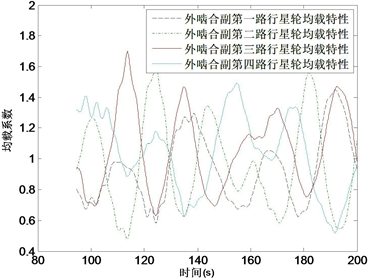Figure 4. Bearing support stiffness of 3 × 106 N/m external meshing vice dynamic load variation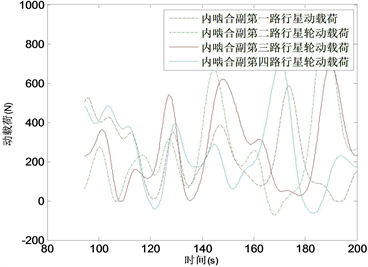Figure 5. Variation of dynamic load on the internal meshing pair when the bearing support stiffness is 3 × 106 N/m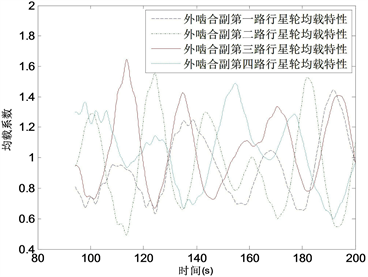Figure 6. Variation of the mean load coefficient when the bearing support stiffness is 3 × 107 N/mFigure 7. Variation of dynamic load on the outer meshing pair when the bearing support stiffness is 3 × 107 N/m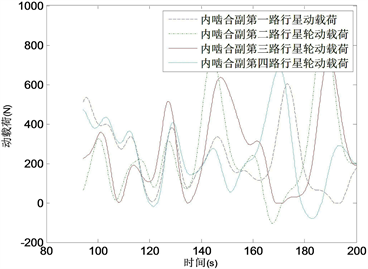Figure 8. Variation of dynamic load on the internal meshing pair when the bearing support stiffness is 3 × 107 N/m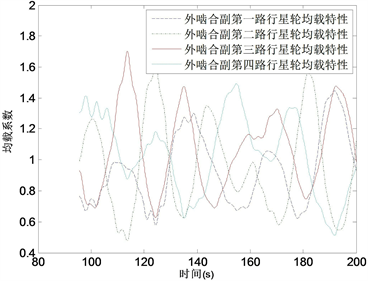Figure 9. Variation of the average load factor when the bearing support stiffness is 3 × 108 N/m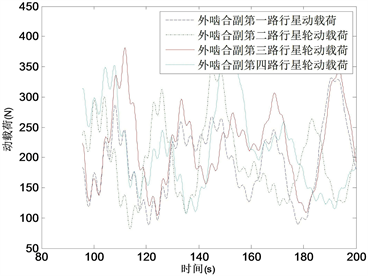Figure 10. Variation of dynamic load of outer meshing vice when bearing support stiffness is 3 × 108 N/m

3. 结论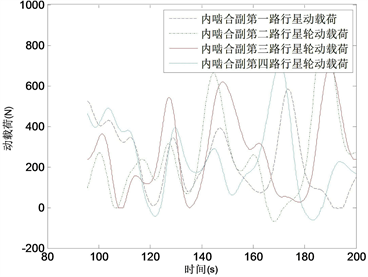Figure 11. Variation of dynamic load on the internal meshing pair when the bearing support stiffness is 3 × 108 N/m

NOTES

*通讯作者。

  李同杰, 靳广虎, 朱如鹏, 等. 高重合度齿轮传动系统的振动参数稳定性[J]. 航空动力学报, 2017, 32(10): 2456-2466.  Huang, K., Yi, Y., Xiong, Y.S., Cheng, Z.B. and Chen, X. (2020) Nonlinear Dynamics Analysis of High Contact Ratio Gears System with Multiple Clearances. Journal of the Brazilian Society of Mechanical Sciences and Engineering, 42, 69-76. https://doi.org/10.1007/s40430-020-2190-0  莫文超, 焦映厚, 陈照波, 等. 人字齿行星传动系统非线性动力学特性分析[J]. 哈尔滨工程大学学报, 2019, 40(10): 1760-1766.  Xiang, L. and Deng, Z.Q. (2020) Dynamical Analysis of Planetary Gear Transmission System under Support Stiffness Effects. International Journal of Bifurcation and Chaos, 30, Article No. 2050080. https://doi.org/10.1142/S0218127420500807  Wang, X. (2019) Nonlinear Dynamics of Planetary Gear Wear in Multistage Gear Transmission System. Journal of Vibroengineering, 21, 1738-1750. https://doi.org/10.21595/jve.2019.20471  张旭东, 杨林杰, 曹延军, 王敬元, 吴鲁纪. 行星齿轮传动系统发展及关键技术研究进展[J]. 化工机械, 2021, 48(6): 803-809.  尚贞, 姚爱民, 李同杰, 马杰, 王娟. 齿侧间隙对行星齿轮传动机构均载特性的影响规律研究[J]. 机械工程与技术, 2017, 6(2): 217-225.